# SSAS Architecture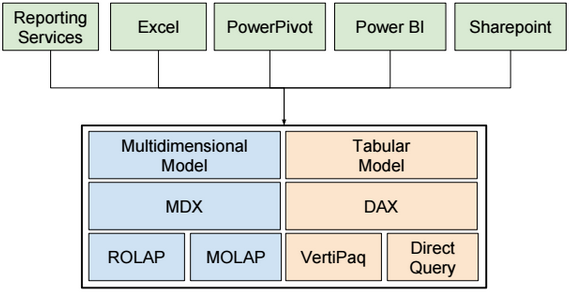# SSAS Server Modes

There are three SSAS Server Modes: Multidimensional, Tabular and Sharepoint.

# SSAS Tabular Architecture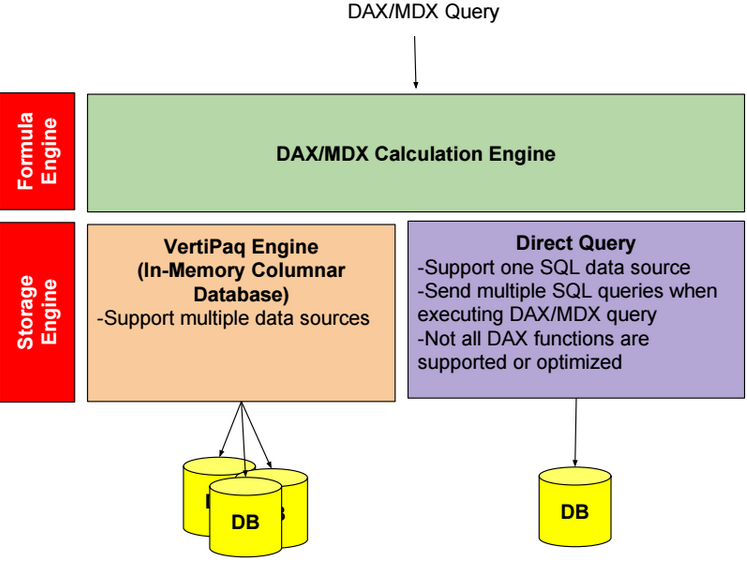# Tabular Model

It’s recommended to use views instead of tables when adding tabular model tables.

View names, column names and tabular table/column names should be user friendly.

For example, use “Customer”, “Sales” and “Quantity” instead of “DimCustomer”, “FactSales” and “Sum of Quantity”.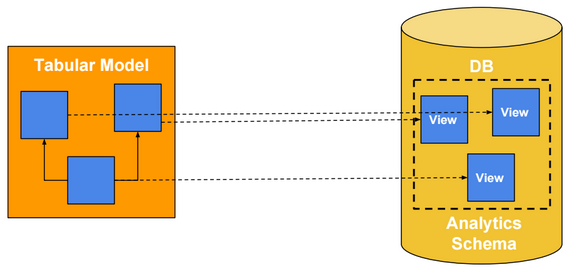# Vertipaq Mode

When processing in-memory partitions, data are loaded and processed by segment. Each segment contains up to 8 millions rows. Each segment is compressed and stored in memory. Uncompressed data are deleted.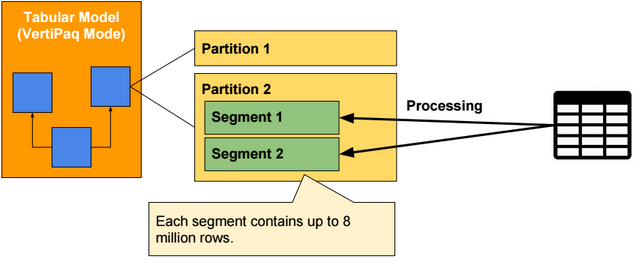Parallel processing of partitions requires more memory than sequential processing.

The number or rows per segment can be changed by specifying a value for the server property DefaultSegmentRowCount.

To monitor memory usage by model object, we can use the following Data Management Views (DMV) tables:

SELECT * FROM \$System.discover_storage_table_columns

# VertiPaq Compression

VertiPaq compression algorithms aim to reduce memory usage of tabular models. There are 3 main encoding algorithms:

Value Encoding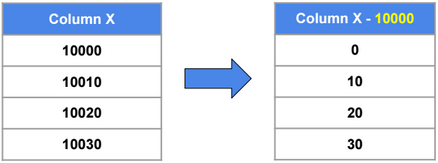Dictionary Encoding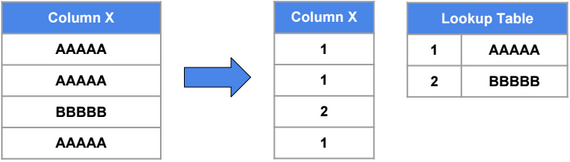Run Length Encoding (RLE)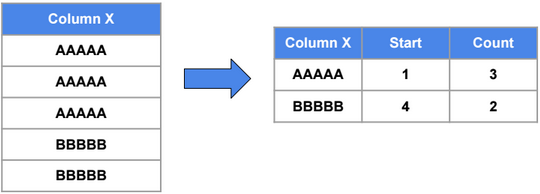# Partitioning

Partitioning does not improve performance, but it simplifies manageability.

It’s recommended to have one or many partitions with size equals to one or many segment sizes (e.g. 16M/24M/… rows).

# Processing

Below SSAS processing options:

 Process Clear Drops all the data in a database, table, or partition. Process Full Loads data into all selected partitions or tables. Any affected calculated columns, relationships, user hierarchies, or internal engine structures (except table dictionaries) are recalculated. Process Data Loads data into a partition or table without recalculating calculated columns. Process Recalc For all tables in the database, recalculates calculated columns, rebuilds relationships, rebuilds user hierarchies, and rebuilds other internal engine structures. Table dictionaries are not affected. Process Add Adds rows to a partition. Any affected calculated columns, relationships, user hierarchies, or internal engine structures (except table dictionaries) are recalculated. Each Process Add creates a new segment. Process Default Loads data into unprocessed partitions or tables. Any affected calculated columns, relationships, user hierarchies, or internal engine structures (except table dictionaries) are recalculated. Process Defrag Optimizes a table dictionary (an internal engine structure) for a given table or for all tables in the database.

Use maxParallelism in the TMSL processing script to limit the number of parallel processing

{

“sequence”: {

maxParallelism” : 2,

“operations” : [ { “refresh” : { “type” : “automatic”, “objects” : [ { “database” : “TabularProject” }]}}]

}.

# Hardware Configuration

CPU and Memory performance are the most important factors when choosing a hardware.

SSAS 2016 SP1 is NUMA-aware. Being NUMA aware means that SSAS tries to reduce at a minimum the usage of the NUMA intersocket connection by preferring the usage of the local RAM bus.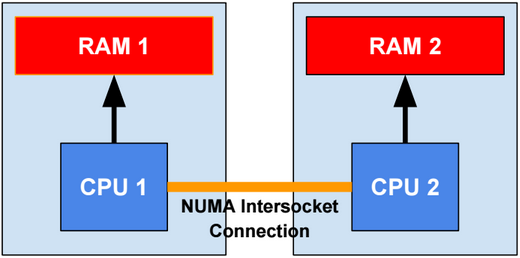# Memory Management

Below server properties related to memory management:

 VertiPaqPagingPolicy Enable/disable memory paging. VertiPaqMemoryLimit (in percentage or in number of bytes) VertiPaq memory limit to store the data (cache excluded). TotalMemoryLimit (in percentage or in number of bytes) Maximum memory that can be used by the SSAS server. LowMemoryLimit (in percentage or in number of bytes) A lower threshold at which the server first begins releasing memory allocated to infrequently used objects. HardMemoryLimit (in percentage or in number of bytes) A threshold at which Analysis Services begins rejecting requests outright due to memory pressure.

We can use Performance Monitor tool to track memory usage.

# Performance Monitoring

The two main tools we can use to monitor performance are Sql Profiler and Extended Events.

When using Extended Events, we need to set AutoRestart to true, so after a restart of the SSAS server, the capture of extended events automatically starts.

# Direct Query Mode

In Direct Query mode, we can create Direct Query partitions and Sample partitions. Direct Query partitions are not stored in-memory. Sample partitions are In-memory partitions, and they are used only during model design. Sample partitions require processing.

From Excel, we can select between using data from Sample partitions or from Direct Query partitions. This option is available when we open Excel from a Tabular model opened in Visual Studio.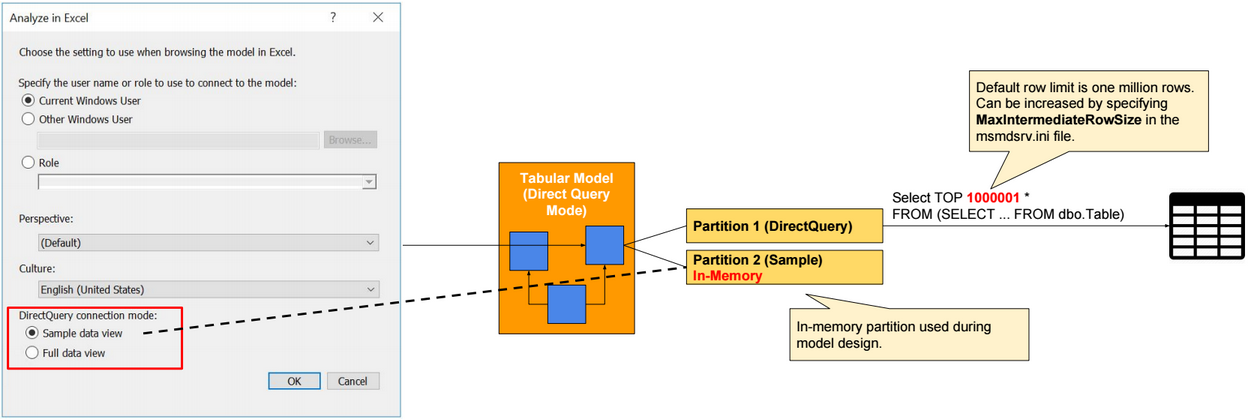In Direct Query mode, if row-level security is defined in data source tables, then we need to configure SSAS to delegate the identity of users when querying data source tables. SSAS can impersonate a Windows user, only when Kerberos Constrained Delegation (KCD) is configured.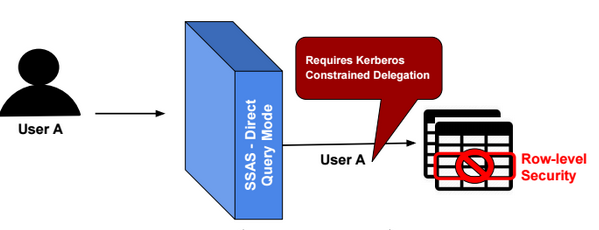More details about Kerberos Constrained Delegation configuration can be found in the following link:

https://docs.microsoft.com/en-us/sql/analysis-services/instances/configure-analysis-services-for-kerberos-constrained-delegation

Row-level security can be configured in data source tables using the following TSQL commands:

CREATE FUNCTION dbo.fn_securitypredicate (@UserId INT)

RETURNS TABLE WITH SCHEMABINDING

AS

RETURN

SELECT 1 as Result WHERE @UserId = USER_NAME() –Return 1 or nothing

CREATE SECURITY POLICY fn_security

ADD FILTER /*OR BLOCK*/ PREDICATE dbo.fn_securitypredicate(UserId) on [dbo].[Table]

# Direct Query Mode Limitations

Below some limitations of Direct Query Mode:

-Hierarchies are not supported in Excel, and they are ignored in MDX queries.

-Can have only one SQL data source.

-Calculated tables cannot be used.

-Some DAX functions (like ALL, CALCULATE, SUM, FILTER,…) are not supported in calculated columns.

-Some DAX functions (like TOPN, GENERATE,..) are not optimized for direct query mode, and they are not converted in corresponding SQL expressions, so they are executed inside the formula engine. As a consequence, they might require transferring large amounts of data between the source database and the SSAS engine.

-Hybrid model (In-Memory partitions + Direct Query partitions) are not supported.

# Role-Based Security

In SSAS Tabular, Roles are additive.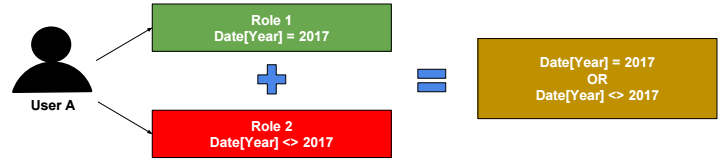Role filters propagate through relationships (from the one side to the many side of one-to-many relationships).

To propagate role filters from the many side to the one side of a one-to-many relationship, we need to set filter direction to “Both Tables”, and check the checkbox “Apply the Filter Direction when using row Level Security”.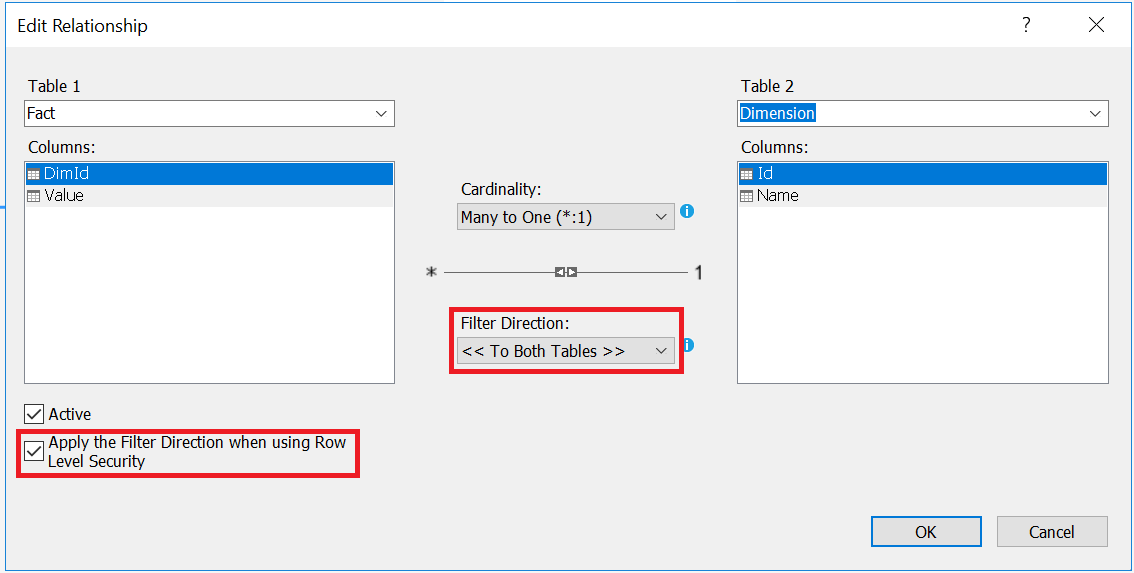Role filters cannot be applied on calculated columns when a Tabular model is In-Memory mode, because calculated columns are evaluated during model processing. In Direct Query mode, role filters are applied when querying source tables.

# Dynamic Security

In the following Tabular model, we use the table “Security” to filter Fact table rows. The DAX filter expression is highlighted in red in the following image.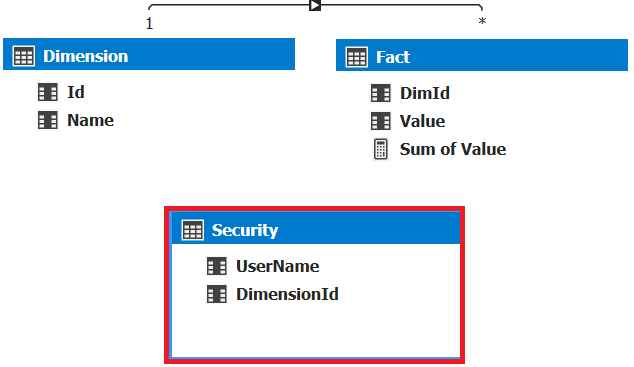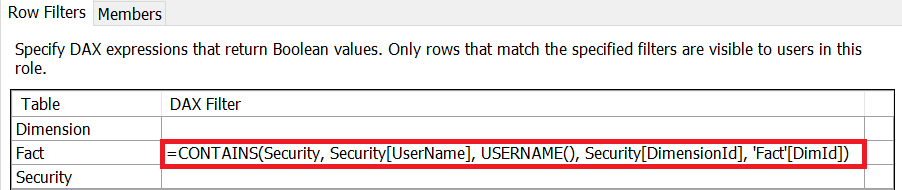We can filter rows using an external parameter defined in the connection string. We can read the value of this parameter using the DAX function CUSTOMDATA.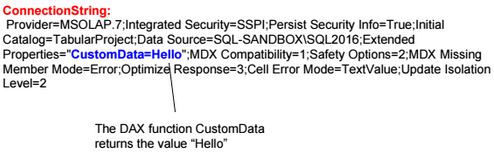# Deployment

There are 4 ways to deploy a tabular model:

1-Using XMLA/TMSL

2-Using SSAS Deployment Wizard

3-Using Synchronize Wizard (Source and destination servers cannot be the same. In general, Synchronize is used to move a database from processing server to main server)

4-Backup/Restore database

.Net libraries

There are two libraries: Analysis Management Objects (AMO) and Tabular Object Model (TOM) that we can use to interact with the SSAS server.

# Recommandations

Avoid large dimensions containing more than one million rows.

More articles and courses related to SSAS Tabular, Power BI and DAX can be found in the SQLBI website: http://www.sqlbi.com/.

# Browse Power BI Desktop Model using Excel or SSMS

Power BI Desktop uses xVelocity technology and loads data into memory. It uses a local instance of SQL Server Analysis Services (SSAS). We can use Excel or SSMS to connect to the local instance. The port used by the instance can be found using the netstat command.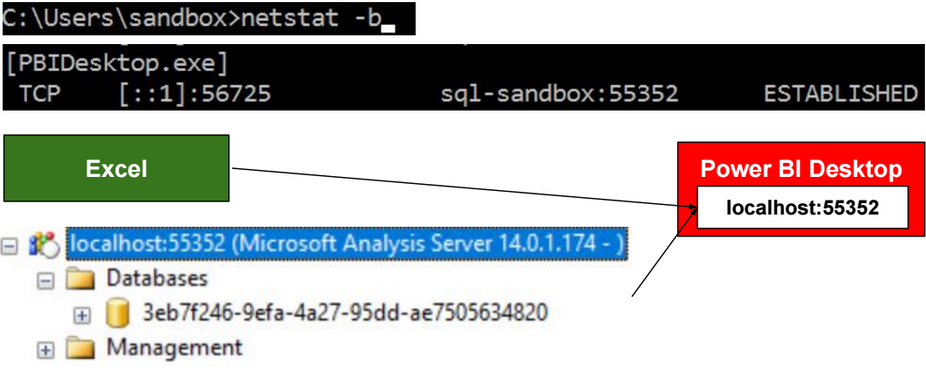# Querying with DAX

Below some DAX queries.

 EVALUATE ‘Dimension’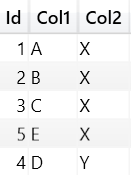EVALUATE ‘Fact’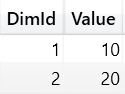EVALUATE ‘Dimension’ ORDER BY ‘Dimension'[Id] START AT 3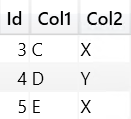EVALUATE CALCULATETABLE(‘Dimension’, ‘Dimension'[Col2]=”X”)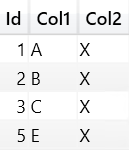EVALUATE ADDCOLUMNS(‘Dimension’, “TotalValue”, [TotalValue])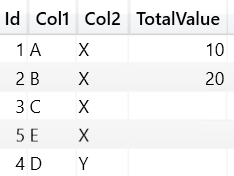EVALUATE SUMMARIZE(‘Dimension’, ‘Dimension'[Col1])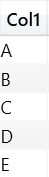EVALUATE SELECTCOLUMNS( ‘Dimension’, “Column 1”,’Dimension'[Col1], “Column 2”,’Dimension'[Col2])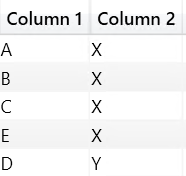EVALUATE SUMMARIZE( ‘Dimension’, ROLLUP(‘Dimension'[Col1]), “TotalValue”, [TotalValue])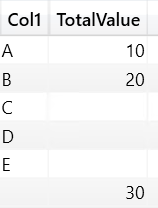EVALUATE CROSSJOIN( ALL(‘Dimension'[Col1]) , ALL(‘Dimension'[Col2]))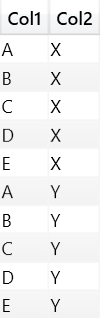EVALUATE GENERATE(‘Dimension’, RELATEDTABLE(‘Fact’))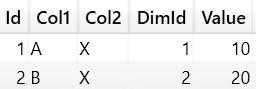EVALUATE ROW(“A”, 1, “B”, 2)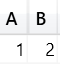EVALUATE TOPN(3,’Dimension’)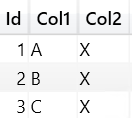EVALUATE UNION(ROW(“A”, 1),ROW(“B”, 2))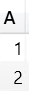EVALUATE INTERSECT(ROW(“A”, 1),ROW(“B”, 1))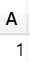EVALUATE SUMMARIZECOLUMNS( ‘Dimension'[Col1], ‘Dimension'[Col2], “TotalValue”, [TotalValue])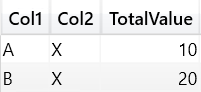EVALUATE GROUPBY( ‘Fact’ ,’Dimension'[id] ,”TotalValue”, SUMX(CURRENTGROUP(), ‘Fact'[Value]))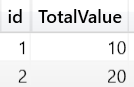WITH MEASURE ‘Fact'[TotalValue1] = SUMX(‘Fact’, ‘Fact'[Value]) SELECT {[TotalValue1]} ON COLUMNS, [Dimension].[Id].MEMBERS ON ROWS FROM [Model]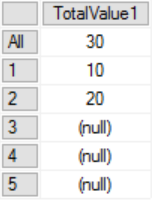DEFINE MEASURE ‘Fact'[TotalValue] = SUMX(‘Fact’, ‘Fact'[Value]) EVALUATE ADDCOLUMNS( SUMMARIZE( ‘Dimension’ ,’Dimension'[Id]) , “TotalValue”, [TotalValue])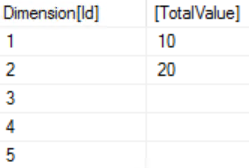# Time Intelligence Functions

The image below shows the output of each Time Intelligence Function when we pass as parameter a column ‘Date’[Date] containing two dates: 2017-12-01 and 2017-12-02.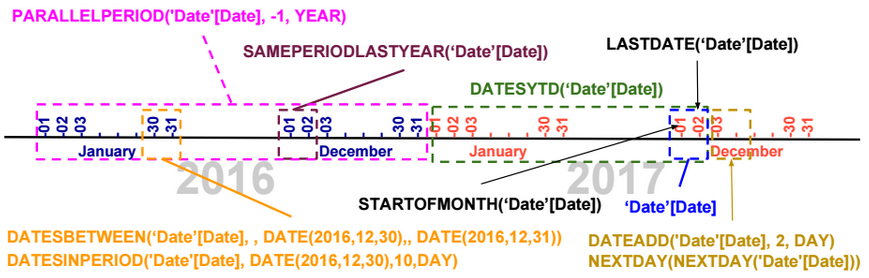Using the CALCULATE function, we can evaluate a measure based on the result of a Time Intelligence function.

CALCULATE([TotalSales], DATESYTD(‘Date’[Date]))

# Last Non Blank Function

LastNonBlank is a DAX function that returns the last value of a column for which an expression is not blank.

The DAX expression below returns the last date the measure Stock is not blank.

LASTNONBLANK(‘Date'[Date], [Stock])

# Calendar Functions

To generate a calendar, we can use the following functions: CALENDAR, CALENDARAUTO.

# Table Functions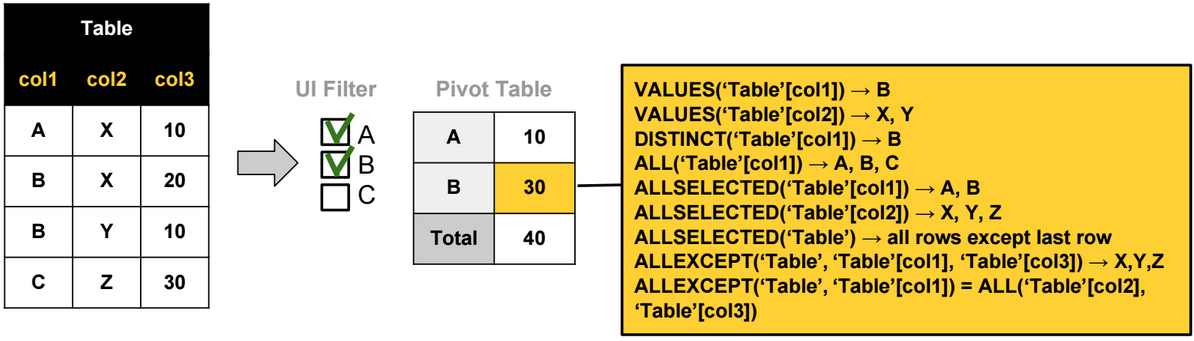The most used Table functions are:

ALL: Ignores any filters that might have been applied on a table or a column.

ALLSELECTED: Ignores any filters that might have been applied on a table or a column, but retains explicit filters (like Excel slicers). (In reality, this function removes all filter contexts generated by context transitions).

DISTINCT: Returns a one-column table that contains the distinct values from the specified column

VALUES: Returns a one-column table that contains the distinct values from the specified table or column.

ALLEXCEPT: Ignores any filters that might have been applied except on the specified columns. For example ALLEXCEPT(‘Table’, Table’[col1]) is equivalent to ALL(‘Table’[col2],‘Table ’[col3]).

ALLNOBLANKROW: Ignores any filters that might have been applied on a table or a column, returns all rows but the blank row, or all distinct values of a column but the blank row.

# Path Functions

Path functions are usually used when defining parent-child hierarchies. Below an example that shows how we can use these functions to create a parent-child hierarchy.

In the table below, we have a parent-child relationship based on two columns: Id and ParentId.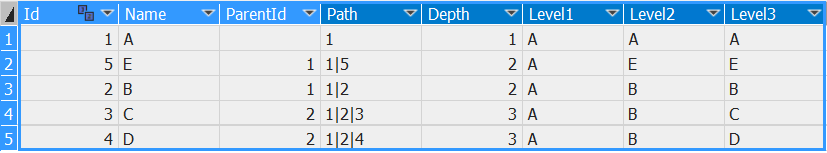The 5 calculated columns on the right are defined using the following DAX expressions:

 Calculated Column DAX Expression Path =PATH([Id],[ParentId]) Depth =PATHLENGTH([Path]) Level1 =IF([Depth] >=1, LOOKUPVALUE([Name],[Id], VALUE(PATHITEM([Path],1,1))), [Name]) Level2 =IF([Depth] >=2, LOOKUPVALUE([Name],[Id], VALUE(PATHITEM([Path],2,1))), [Name]) Level3 =IF([Depth] >=3, LOOKUPVALUE([Name],[Id], VALUE(PATHITEM([Path],3,1))), [Name])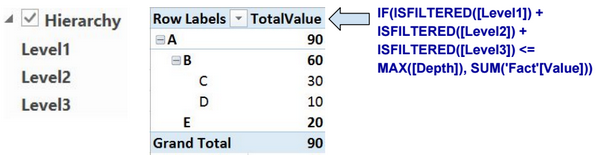We use the columns Level1, Level2, and Level3 to define the parent-child hierarchy.

# LookUpValue Function

LookupValue function retrieves a lookup value from a lookup table.

# Count Functions

COUNT: Count numeric values.

COUNTA: Count rows different than blank.

COUNTBLANK: Count rows equal to blank.

COUNTROWS: COUNTA + COUNTBLANK.

DISTINCTCOUNT: Distinct count including Blank values.

# Other Functions

ISEMPTY

IFERROR, ISERROR

LEN, LEFT, RIGHT, SEARCH, SUBSTITUTE, REPLACE, FORMAT

DIVIDE

SWITCH

ISBLANK, ISTEXT, ISNONTEXT, ISNUMBER

CONCATENATEX

RANKX

ISFILTERED, ISCROSSFILTERED

HASONEVALUE

FLOOR, TRUNC, ROUNDDOWN, CEILING, MROUND, ROUNDUP

YEAR, MONTH, DAY

More details about these functions can be found here: https://msdn.microsoft.com/en-us/library/ee634396.aspx

# Evaluation Contexts

There are 3 types of evaluation contexts.

 Row Context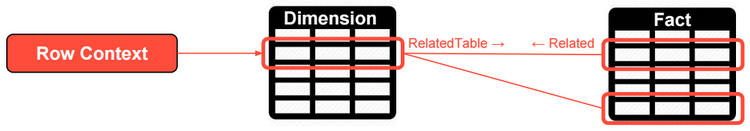Row context includes the values from all columns in a row and related rows. Row contexts doesn’t propagate through relationships (from the one side to the many side of one-to-many relationships). Filter Context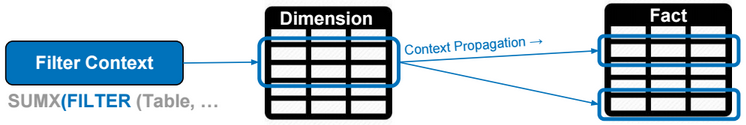Filter context specifies filter constraints on the set of values allowed in a column or a table. Filter context propagates through relationships. Query Context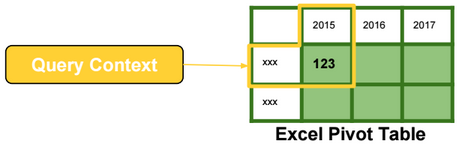Query context is the filtering applied to a cell in the pivot table. The filter context and the query context are almost identical in their effects.

# X Functions

X Functions are iterators. They evaluate expressions by iterating over table rows.

The following DAX expression is evaluated by running a loop over rows of the Sales table.

[TotalSales] = SUMX (Sales, Sales[Quantity] * Sales[Net Price])Rows with values not allowed in the filter/query context are ignored.

# Context Transition

Context transition is the transformation of row contexts into an equivalent filter context.

For example, to evaluate the DAX expression below, we need to iterate over rows in the Date table. For each Date row, we need to evaluate the measure TotalSales, and then calculate the average of daily sales.

AvgDailySales = AverageX(Date, [TotalSales])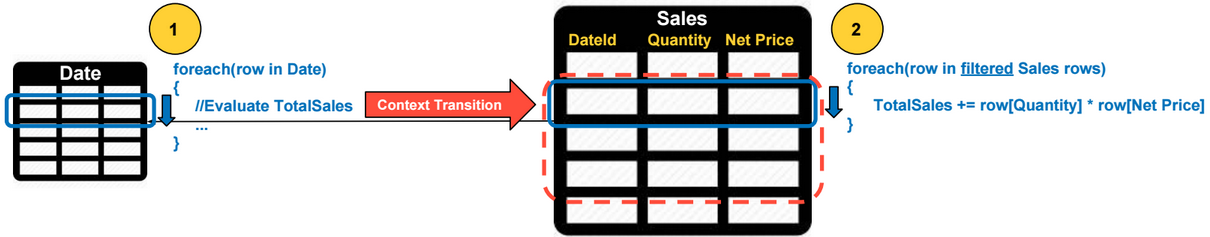For each Date row, a context transition occurs, and values from all columns in a Date row are used as filters when evaluating the TotalSales. Context transition occurs because TotalSales is a measure.

Context transition automatically occurs when the input expression of the X function is a measure, otherwise we need to use the CALCULATE function to perform context transitions like in the following example:

AvgDailySales = AverageX(Date, CALCULATE(SUMX(Sales, Sales[Quantity] * Sales[Net Price])))

# Filter Context Intersection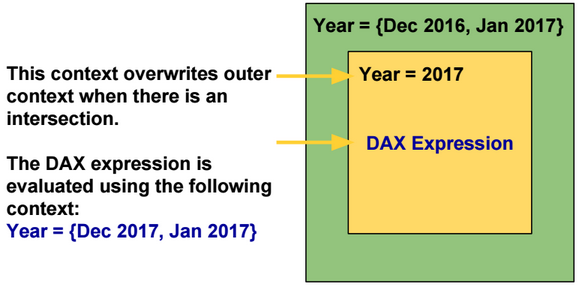By default inner filter contexts overwrite outer filter contexts when there is an intersection.

The KEEPFILTERS function modifies this behavior by using Intersect instead of Overwrite.

 Overwrite Intersect -Outer Filter Context: {Dec 2016, Jan 2017} -Inner Filter Context: {Year = 2017} {Dec 2017, Jan 2017} {Jan 2017}

# Filter Function

Filter function is also an iterator. It filters rows based on a list of input conditions.

# Calculate Function

CALCULATE evaluates an expression in a context that is modified by the specified filters.

The following DAX expressions are equivalent. The filter expression of the calculate function can be a table.

[TotalSales2017] = CALCULATE([TotalSales], ‘Date'[Year] = 2017)

[TotalSales2017] = CALCULATE([TotalSales], FILTER(ALL(‘Date'[Year]), ‘Date'[Year] = 2017)

CALCULATE always performs context transition by transforming row context to filter context. In the DAX expressions above, the row context is empty.

# Context Propagation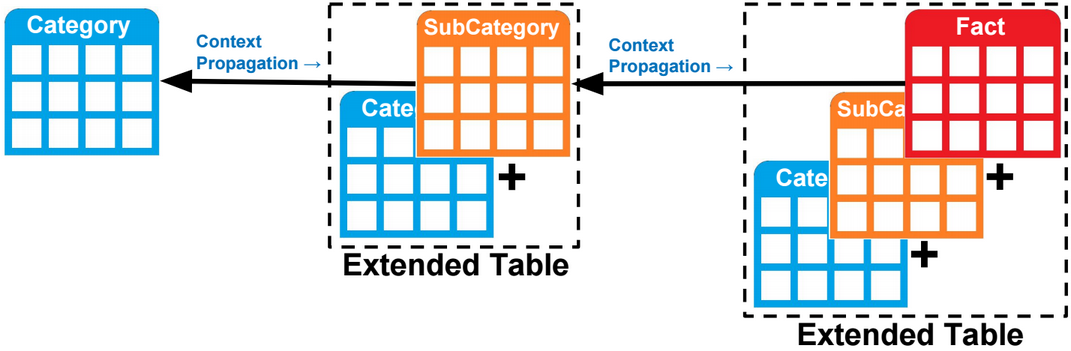Filter context propagates (by default) from the one side to the many side of a one-to-many relationship.

Filter context can also propagates from the many side to the one side of a one-to-many relationship. For example, the DAX expression below counts the number of rows in the category table when one or many sub categories are selected.

CALCULATE(COUNTROWS(‘Category’), ‘SubCategory‘)

In reality, there is no context propagation, but instead, tables are extended to include all columns in related tables, and filtering a column instantly filters all extended tables referencing that column.

To propagate a filter context from the many side to the one side of a one-to-many relationship, we need to use the Calculate function and specify as parameter the many side table.

ALL function removes all filters defined on a table and related tables. To keep filters defined on a related table, we need to specify that related table as a parameter to the calculate function.

CALCULATE(COUNTROWS(‘SubCategory’), ALL(‘SubCategory’), ‘Category’)

# Relationships

Relationships in a model could be one-way or two-way. A relationship is deactivated if it’s causing ambiguity in the model.

The model below shows two paths to propagate contexts from the table on the left to the one on the right. This model is not supported and one of the relationships should be deactivated.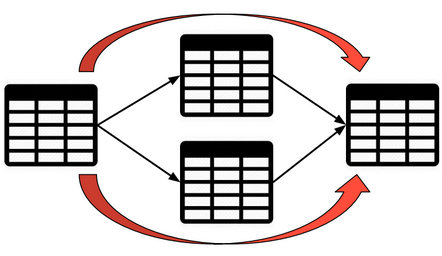To activate and use a relationship when evaluating a DAX expression, we can use the USERELATIONSHIP function.

CALCULATE([TotalSales], USERELATIONSHIP(Sales[OrderDate],Date[Id]))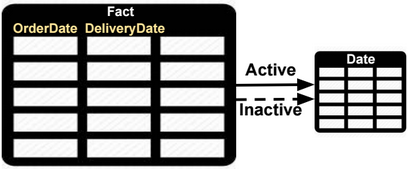To change the filter direction of a relationship, we can use the CROSSFILTER function.

CALCULATE([TotalSales], CROSSFILTER(Sales[OrderDate],Date[Id], BOTH))

# Many-to-many Relationships

Relationships in a model could be one-way or two-way. In a two-way relationship, filter contexts propagate in both directions.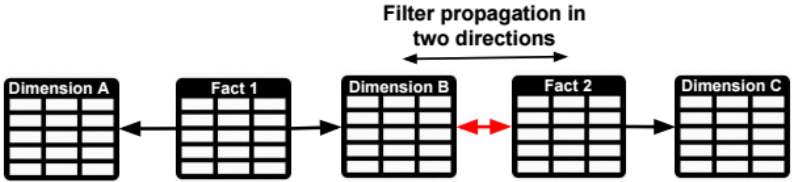If the model doesn’t support two-way relationships, then we need to specify the many-to-many (M2M) fact table as parameter of the Calculate function.

For example, if we want to propagate a filter on Dimension C to Fact1, we only need to specify Fact 2 as parameter of the Calculate function. The extended Fact 2 table includes all columns of related tables (Dimensions B and C), and filtering Fact 2 table also filters Dimension B columns that are in the extended Fact 2 table.

CALCULATE([TotalSales], Fact2)

# Virtual Relationships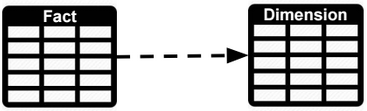We can simulate a relationship between two tables using DAX. For example to calculate the TotalSales for values in a dimension table we can use the following formula:

CALCULATE([TotalSales], FILTER(‘Fact’, CONTAINS(‘Dimension’, ‘Dimension'[Id], ‘Fact'[Id])))

# Calculated Column vs Measure

 Calculated column Consume more memory. The filter context is always empty when defining a Calculated Column. Measure Consume more CPU. The filter context is automatically applied on measures.